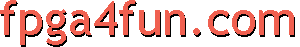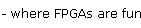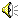## Music box 3 - Playing notes

Now we'd like to play a tune. We need a way to play notes, like on a keyboard.If we use 6 bits to encode a note, we can get 64 notes. There are 12 notes per octaves, so 64 notes gives us over 5 octaves, more than enough for a little tune.

##### Step 1.
To play a range of increasing notes, we instantiate a 28 bits counter, from which we extract the 6 most significant bits, to give us the 6 bits of the note we want to play.

 reg [27:0] tone; always @(posedge clk) tone <= tone+1; wire [5:0] fullnote = tone[27:22];

With a 25Mhz clock, each note lasts 167ms and it takes 10.6s to play all 64 notes.
##### Step 2.
We divide the "fullnote" by 12. That gives us the octave (5 octaves, so 3 bits are enough, since it goes from 0 to 4) and the note (from 0 to 11, so 4 bits).

 wire [2:0] octave; wire [3:0] note; divide_by12 divby12(.numer(fullnote[5:0]), .quotient(octave), .remain(note));

You can see that we instantiate a sub-module called "divide_by12" which takes care of the division. Details are shown later.
##### Step 3.
To go from one octave to the next, frequency is multiplied by "2". Easy to do in hardware, we do that in step 4.
To go from one note to the next, frequency is multiplied by "1.0594". Not really easy to do in hardware. So we use a look-up table with pre-calculated values.

We divide the main clock by 512 for note A, by 483 for note A#, by 456 for note B... Remember, dividing by a lower value gives us a higher frequency/higher note.

 always @(note) case(note)   0: clkdivider = 512-1; // A   1: clkdivider = 483-1; // A#/Bb   2: clkdivider = 456-1; // B   3: clkdivider = 431-1; // C   4: clkdivider = 406-1; // C#/Db   5: clkdivider = 384-1; // D   6: clkdivider = 362-1; // D#/Eb   7: clkdivider = 342-1; // E   8: clkdivider = 323-1; // F   9: clkdivider = 304-1; // F#/Gb   10: clkdivider = 287-1; // G   11: clkdivider = 271-1; // G#/Ab   12: clkdivider = 0; // should never happen   13: clkdivider = 0; // should never happen   14: clkdivider = 0; // should never happen   15: clkdivider = 0; // should never happen endcase always @(posedge clk) if(counter_note==0) counter_note <= clkdivider; else counter_note <= counter_note-1;

Every time "counter_note" equals 0, that gives us a tick for the next stage: the octave divider.
##### Step 4.
Ok, we need now to take care of the octave.
For the lowest octave, we divide "counter_note" by 256. For octave 1, we divide by 128... and so on...

 reg [7:0] counter_octave; always @(posedge clk) if(counter_note==0) begin  if(counter_octave==0)   counter_octave <= (octave==0?255:octave==1?127:octave==2?63:octave==3?31:octave==4?15:7);  else   counter_octave <= counter_octave-1; end reg speaker; always @(posedge clk) if(counter_note==0 && counter_octave==0) speaker <= ~speaker;

The full code is here:

 module music(clk, speaker); input clk; output speaker; reg [27:0] tone; always @(posedge clk) tone <= tone+1; wire [5:0] fullnote = tone[27:22]; wire [2:0] octave; wire [3:0] note; divide_by12 divby12(.numer(fullnote[5:0]), .quotient(octave), .remain(note)); reg [8:0] clkdivider; always @(note) case(note)   0: clkdivider = 512-1; // A   1: clkdivider = 483-1; // A#/Bb   2: clkdivider = 456-1; // B   3: clkdivider = 431-1; // C   4: clkdivider = 406-1; // C#/Db   5: clkdivider = 384-1; // D   6: clkdivider = 362-1; // D#/Eb   7: clkdivider = 342-1; // E   8: clkdivider = 323-1; // F   9: clkdivider = 304-1; // F#/Gb   10: clkdivider = 287-1; // G   11: clkdivider = 271-1; // G#/Ab   12: clkdivider = 0; // should never happen   13: clkdivider = 0; // should never happen   14: clkdivider = 0; // should never happen   15: clkdivider = 0; // should never happen endcase reg [8:0] counter_note; always @(posedge clk) if(counter_note==0) counter_note <= clkdivider; else counter_note <= counter_note-1; reg [7:0] counter_octave; always @(posedge clk) if(counter_note==0) begin  if(counter_octave==0)   counter_octave <= (octave==0?255:octave==1?127:octave==2?63:octave==3?31:octave==4?15:7);  else   counter_octave <= counter_octave-1; end reg speaker; always @(posedge clk) if(counter_note==0 && counter_octave==0) speaker <= ~speaker; endmodule
##### Divide by 12:

The "divide by 12" module takes a variable 6 bits value (numerator) and divides it by the fixed value 12 (denominator). That gives us a 3 bits quotient (0..5) and a 4 bits remainder (0..11). To divide by 12, it is easier to divide by 4 first, then by 3. Dividing by 4 is trivial: we remove 2 bits out of the numerator, and copy it to the remainder. So we are left with 6-2=4 bits to divide by the value "3", which we do with a lookup table.

 module divide_by12(numer, quotient, remain); input [5:0] numer; output [2:0] quotient; output [3:0] remain; reg [2:0] quotient; reg [3:0] remain_bit3_bit2; assign remain = {remain_bit3_bit2, numer[1:0]}; // the first 2 bits are copied through always @(numer[5:2]) // and just do a divide by "3" on the remaining bits case(numer[5:2])    0: begin quotient=0; remain_bit3_bit2=0; end    1: begin quotient=0; remain_bit3_bit2=1; end    2: begin quotient=0; remain_bit3_bit2=2; end    3: begin quotient=1; remain_bit3_bit2=0; end    4: begin quotient=1; remain_bit3_bit2=1; end    5: begin quotient=1; remain_bit3_bit2=2; end    6: begin quotient=2; remain_bit3_bit2=0; end    7: begin quotient=2; remain_bit3_bit2=1; end    8: begin quotient=2; remain_bit3_bit2=2; end    9: begin quotient=3; remain_bit3_bit2=0; end  10: begin quotient=3; remain_bit3_bit2=1; end  11: begin quotient=3; remain_bit3_bit2=2; end  12: begin quotient=4; remain_bit3_bit2=0; end  13: begin quotient=4; remain_bit3_bit2=1; end  14: begin quotient=4; remain_bit3_bit2=2; end  15: begin quotient=5; remain_bit3_bit2=0; end endcase endmodule